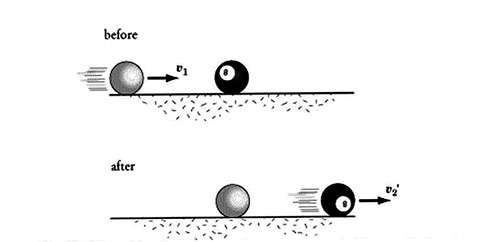Physics

# What is Collision?

A collision between two particles is said to occur if they physically strike against each other or if the path of the motion of one is influenced by the other. In physics, the term collision does not necessarily mean that a particle actually strikes. In fact, two particles may not even touch each other and yet they are said to collide if one particle influences the motion of the other.When two bodies collide, each body exerts a force on the other. The two forces are exerted simultaneously for an equal but short interval of time. According to Newton’s third law of motion, each body exerts an equal and opposite force on the other at each instant of collision. During a collision, the two fundamental conservation laws namely, the law of conservation of momentum and that of energy are obeyed and these laws can be used to determine the velocities of the bodies after a collision. Collisions are divided into two types : (i) elastic collision and (ii) inelastic collision